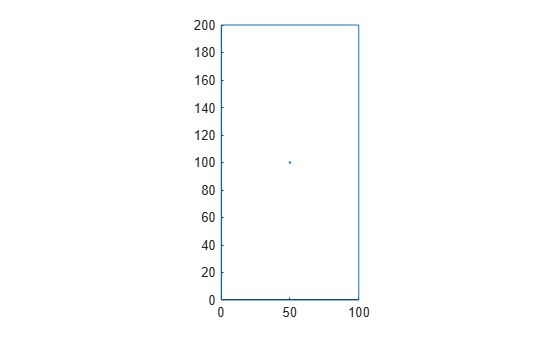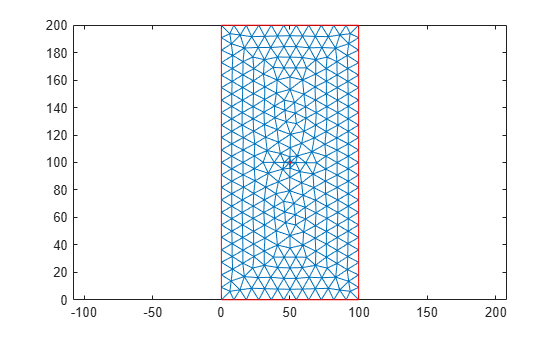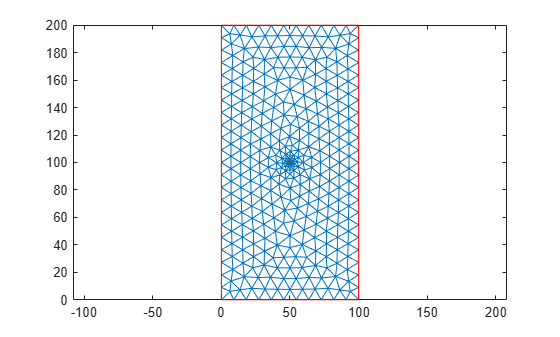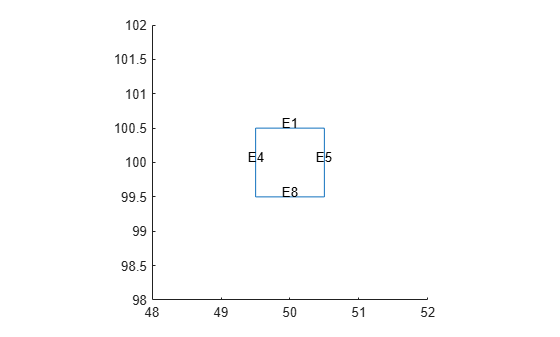# Generate Mesh

The `generateMesh` function creates a triangular mesh for a 2-D geometry and a tetrahedral mesh for a 3-D geometry. By default, the mesh generator uses internal algorithms to choose suitable sizing parameters for a particular geometry. You also can use additional arguments to specify the following parameters explicitly:

• Target maximum mesh edge length, which is an approximate upper bound on the mesh edge lengths. Note that occasionally, some elements can have edges longer than this parameter.

• Target minimum mesh edge length, which is an approximate lower bound on the mesh edge lengths. Note that occasionally, some elements can have edges shorter than this parameter.

• Mesh growth rate, which is the rate at which the mesh size increases away from the small parts of the geometry. The value must be between 1 and 2. This ratio corresponds to the edge length of two successive elements. The default value is 1.5, that is, the mesh size increases by 50%.

• Quadratic or linear geometric order. A quadratic element has nodes at its corners and edge centers, while a linear element has nodes only at its corners.

Create a PDE model.

`model = createpde;`

Include and plot the following geometry.

```importGeometry(model,"PlateSquareHolePlanar.stl"); pdegplot(model)```Generate a default mesh. For this geometry, the default target maximum and minimum mesh edge lengths are 8.9443 and 4.4721, respectively.

`mesh_default = generateMesh(model)`
```mesh_default = FEMesh with properties: Nodes: [2x1218 double] Elements: [6x574 double] MaxElementSize: 8.9443 MinElementSize: 4.4721 MeshGradation: 1.5000 GeometricOrder: 'quadratic' ```

View the mesh.

```figure pdemesh(mesh_default)```For comparison, create a mesh with the target maximum element edge length of 20.

`mesh_Hmax = generateMesh(model,"Hmax",20)`
```mesh_Hmax = FEMesh with properties: Nodes: [2x286 double] Elements: [6x126 double] MaxElementSize: 20 MinElementSize: 10 MeshGradation: 1.5000 GeometricOrder: 'quadratic' ```
```figure pdemesh(mesh_Hmax)```Now create a mesh with the target minimum element edge length of 0.5.

`mesh_Hmin = generateMesh(model,"Hmin",0.5)`
```mesh_Hmin = FEMesh with properties: Nodes: [2x1378 double] Elements: [6x654 double] MaxElementSize: 8.9443 MinElementSize: 0.5000 MeshGradation: 1.5000 GeometricOrder: 'quadratic' ```
```figure pdemesh(mesh_Hmin)```Create a mesh, specifying both the maximum and minimum element edge lengths instead of using the default values.

```mesh_HminHmax = generateMesh(model,"Hmax",20, ... "Hmin",0.5)```
```mesh_HminHmax = FEMesh with properties: Nodes: [2x458 double] Elements: [6x212 double] MaxElementSize: 20 MinElementSize: 0.5000 MeshGradation: 1.5000 GeometricOrder: 'quadratic' ```

View the mesh.

```figure pdemesh(mesh_HminHmax)```Create a mesh with the same maximum and minimum element edge lengths, but with the growth rate of 1.9 instead of the default value of 1.5.

```mesh_Hgrad = generateMesh(model,"Hmax",20, ... "Hmin",0.5, ... "Hgrad",1.9)```
```mesh_Hgrad = FEMesh with properties: Nodes: [2x390 double] Elements: [6x178 double] MaxElementSize: 20 MinElementSize: 0.5000 MeshGradation: 1.9000 GeometricOrder: 'quadratic' ```
```figure pdemesh(mesh_Hgrad)```You also can choose the geometric order of the mesh. The toolbox can generate meshes made up of quadratic or linear elements. By default, it uses quadratic meshes, which have nodes at both the edge centers and corner nodes.

```mesh_quadratic = generateMesh(model,"Hmax",50); figure pdemesh(mesh_quadratic,"NodeLabels","on") hold on plot(mesh_quadratic.Nodes(1,:), ... mesh_quadratic.Nodes(2,:), ... "ok","MarkerFaceColor","g") ```To save memory or solve a 2-D problem using a legacy solver, override the default quadratic geometric order. Legacy PDE solvers require linear triangular meshes for 2-D geometries.

```mesh_linear = generateMesh(model, ... "Hmax",50, ... "GeometricOrder","linear"); figure pdemesh(mesh_linear,"NodeLabels","on") hold on plot(mesh_linear.Nodes(1,:), ... mesh_linear.Nodes(2,:), ... "ok","MarkerFaceColor","g") ```